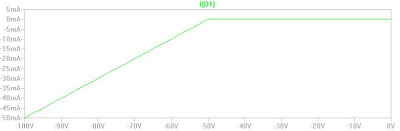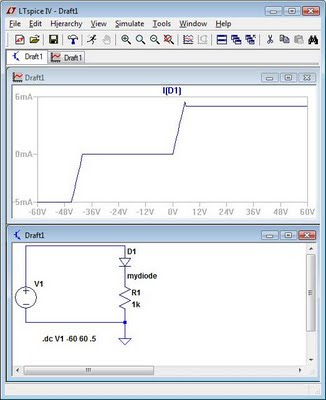## Thursday, November 19, 2009

### Modeling Temperature in LTSpice - Part I

We can model temperature behavior in LTSpice using the built in step function.

To model a list of temps, insert the spice directive ".step temp list "
example: .step temp list -25 -10 10 20 25 50 75

To model a sweep, insert the spice directive ".step temp "
example: .step temp -55 125 25

The following example models a simple transistor amplifier from -55C to 125C in 25C steps.The transient analysis below shows the source current over temperature.## Saturday, November 7, 2009

### Modeling Diode Reverse Breakdown Voltages in LTSpice

The included models in LTSpice do not include reverse breakdown. In order to model the reverse breakdown "vrev" can be specified in the diode model. For this example, create a new schematic and call the diode "mydiode". Insert the .model directive to add the reverse breakdown. Below, I used all the standard parameters for the 1N4148 and added vrev=50. The vrev specifies the reverse breakdown at -50V. Many other diode properties can be defined in the model including the non-linear characteristics of the breakdown.Forward and reverse breakdown and current limitations can be modeled by using a more sophisticated model. Using the model below, forward and reverse current limiting can be observed.

.model mydiode D(vrev=40 Ibv=20nA BV=40 Ibv=1e-10 Is=1e-7 Rs=10 Tt=2n Ilimit=5mA Vpk=40 Revilimit=5mA)